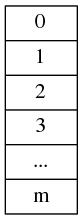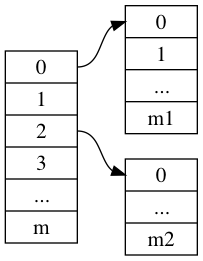# Before

Thanks for inviting me to answer the question: “Design a HashMap”. The description of the question is here:

All of us knows “HashMap”. It is not “HashTable” see Figure 1.1. It means there does not exist a big array to store all of data.Figure 1.1 hashtable

Compute hash(key) and put the value into array[hash(key)]. If array[hash(key)] is stored by other data, you must not put it into another slot. See Figure 1.2. You should create space for storing your new data.Figure 1.2 hashmap

# Complexity

How to design it? We should have a hash function. And create an array/list called slot/bucket to store pointers/references which point to real data. Simple? But we should not implement the code immediately. Consider the functions and complexity first.

I assume complexity of hash function is O(hash). And the complexity of Operator is T(n). It means doing the operator n times. We look at put function. O(hash) often is done in constant time as O(1). But in special case like hash(Big Integer or Long String), the complexity of hash function will be O(x), x is related with the length of Big Integer or Long String. Em…Those are extreme cases, and we don’t care! So we just consider O(hash) as O(1).

So what’s the O(insert into new space) ? I don’t know. If it is a single linklist, it will be O(1). We can insert the data into the head of linklist. But if we use other data structures? I will not discuss here. I should list other operaters first.

We assume our hash function is extreme good. The function will decentralize keys homogeneously. According to pigeon hole principle, the length of every space is no more than (N/m + 1). N is the total number of your data. m is the number of slots.

and containsKey

Emm…The complexity of containsKey is similar with that of get(key). contain(key) is equal with get(key) ? Maybe.
and next is containsValue

In the simple hashmap, there dose not exist value -> key mappings. so the complexity is O(n). We should iterator all values to find the target one.

And next is remove

If in put(key,value), we use single linklist and insert key-value into head every time. We find the key-value is O(N/m +1) and remove it is O(1). So

# Optimize?

Can we use other data structures to optimize some operation? Yes. But we should consider other factors. like More complex, more bugs. and Effect of optimizing operation. For example, we consider the operation containsValue(value) is bad performance O(n). We can use another kind of hashmap structure to store value -> key mapping. So if we call containsValue(value), we will first call getKey(value) to get key, and then call get(key) to find the value. It sounds good! But how often we will call containsValue(value)? Maybe in every 1000 operations, only 1 operation is containsValue(value) and 999 are containsKey/put/get/remove. There is a little better effect vs more complex code. Which do you choose? And if you don’t optimize containsValue, will user think your application is too slow to use?